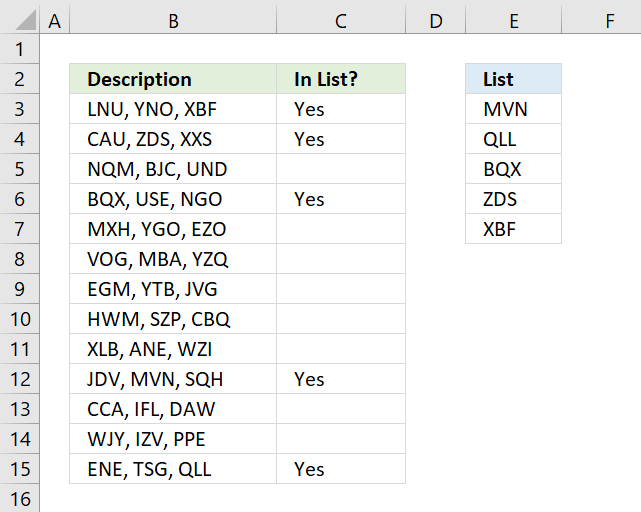Author: Oscar Cronquist Article last updated on March 25, 2018The array formula in cell C3 checks if cell B3 contains at least one of the values in List (E3:E7), it returns "Yes" if any of the values are found in column B and returns nothing if cell contains none of the values.

Example, cell B3 contains XBF which is found in cell E7. Cell B4 contains text ZDS found in cell E6. Cell C5 contains no values in list.

=IF(OR(COUNTIF(B3,"*"&\$E\$3:\$E\$7&"*")), "Yes", "")

The COUNTIF function has ampersands concatenated to cell range E3:E7 which makes it count the cell if it contains a value in the list.

COUNTIF(B3,"*"&\$E\$3:\$E\$7&"*")

becomes

COUNTIF("LNU, YNO, XBF", {"*MVN*"; "*QLL*"; "*BQX*"; "*ZDS*"; "*XBF*"})

and returns this array

{0;0;0;0;1}

which tells us that the last value in list is found in cell B3. The OR function returns TRUE if at least one of the values in the array is 1.

OR({0;0;0;0;1})

returns TRUE.

The IF function then returns "Yes" if logical test is TRUE and nothing if logical test is FALSE.

IF(TRUE, "Yes", "")

returns "Yes" in cell B3.

To enter an array formula press and hold CTRL + SHIFT simultaneously, then press Enter once. Release all keys.

The formula bar now shows the formula enclosed with curly brackets telling you that you entered the formula successfully. Don't enter the curly brackets yourself.

### Alternative formula

The following formula is quite similar to the formula above except that it is a regular formula and it has an additional INDEX function.

=IF(OR(INDEX(COUNTIF(B3,"*"&\$E\$3:\$E\$7&"*"),)), "Yes", "")